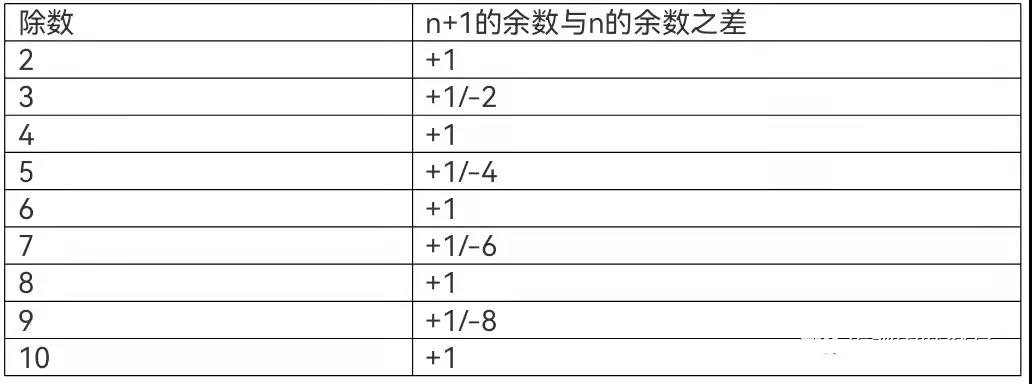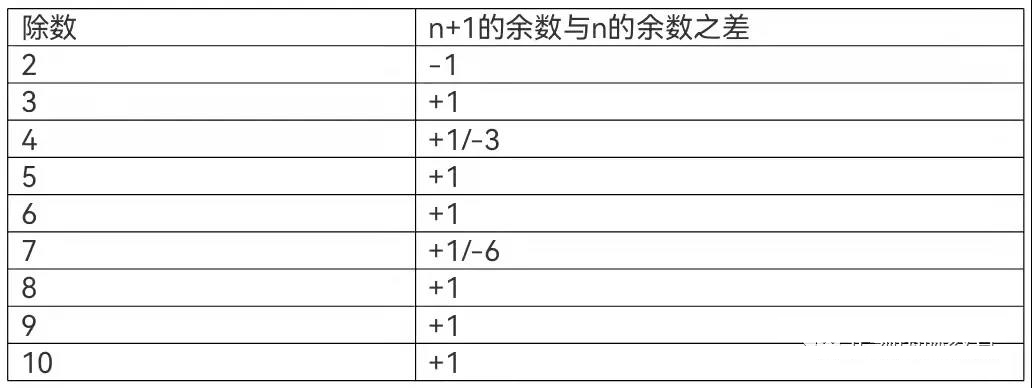# 2021 AMC12B第25题解析！AMC12B最后一题解析

2021 AMC12B第25题解析！AMC10/12考试已经结束了，大家当前需要将重点放在试题解析上，为之后的考试做准备，下面就一起来看看最后一题是什么，以及我们应该如何解析。

Question 25

For n a positive integer, let R(n) be the sum of remainders when n is divided by 2,3,4,5,6,7,8,9,and10. For example, R(15)=1+0+3+0+3+1+7+6+5=26. How many two-digit positive integers n satisfy R(n)=R(n+1)?